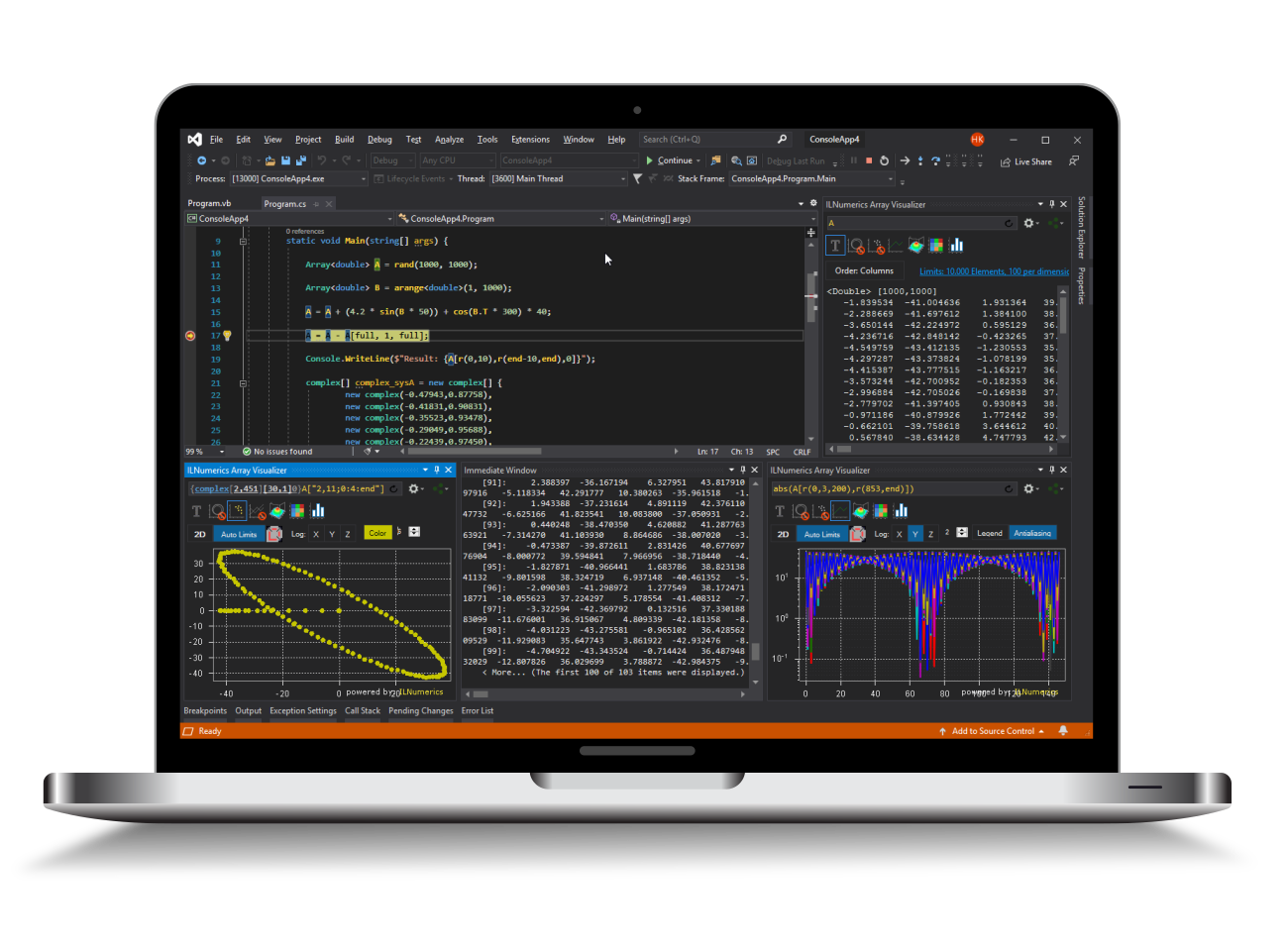Industrial Data Science
in C# and .NET:
Simple. Fast. Reliable.ILNumerics - Technical Computing

Modern High Performance Tools for Technical

Computing and Visualization in Industry and Science

tgt

# Singular Value Decomposition (SVD) in .NET (C#, Visual Basic)

The singular value decomoposition or SVD decomposes a real or complex m x n matrix A into three matrices

$$A = U \cdot S \cdot V^*$$

where $U^*$ indicates transpose and complex conjugation of $U$. The matrices $U$ and $V$ are complex orthonormal matrices, which means that the column and row vectors are pairwise orthonormal. As a consequence the inverse of $U$ and $V$ are $U^*$ and $V^*$, respectively.

$S$ is a diagonal matrix with the so-called singular values as entries. The columns of $U$ are the left singular vectors and the columns of $V$ are the right singular values.

## Calculating the Singular Value Decomposition (SVD) in .NET (C#, Visual Basic)

ILNumerics provides routines to calculate the singular value decomposition (SVD) for single and double precision real and complex matrices. Various overloads of svd are available. The simplest just calculates the singular values

## VB code for Singular Value Decomposition

If all matrices are needed you can call